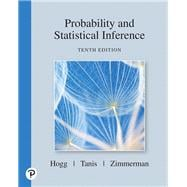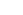# Probability and Statistical Inference

• ISBN 13:

• ISBN 10:

## 013518939X

• Edition: 10th
• Format: Hardcover
• Publisher: Pearson

Note: Not guaranteed to come with supplemental materials (access cards, study guides, lab manuals, CDs, etc.)

Extend Your Rental at Any Time

Rental Options

List Price \$226.65 SaveFree Shipping On Every Order
In Stock Usually Ships Within 24-48 hours.

### Summary

For one- or two-semester courses in Probability, Probability & Statistics, or Mathematical Statistics.

An authoritative introduction to an in-demand field

Advances in computing technology – particularly in science and business – have increased the need for more statistical scientists to examine the huge amount of data being collected. Written by veteran statisticians,  Probability and Statistical Inference, 10th Edition emphasizes the existence of variation in almost every process, and how the study of probability and statistics helps us understand this variation.

This applied introduction to probability and statistics reinforces basic mathematical concepts with numerous real-world examples and applications to illustrate the relevance of key concepts. It is designed for a two-semester course, but it can be adapted for a one-semester course. A good calculus background is needed, but no previous study of probability or statistics is required.

013518939X / 9780135189399  PROBABILITY AND STATISTICAL INFERENCE, 10/e